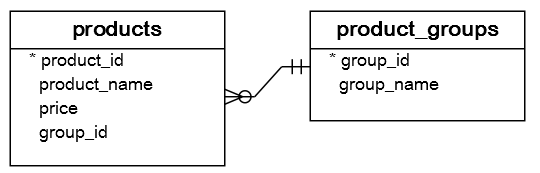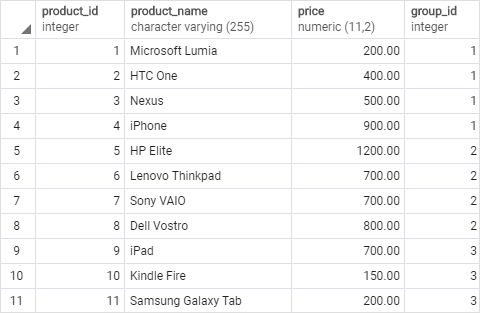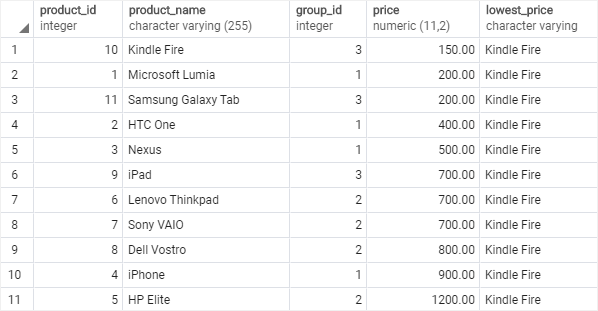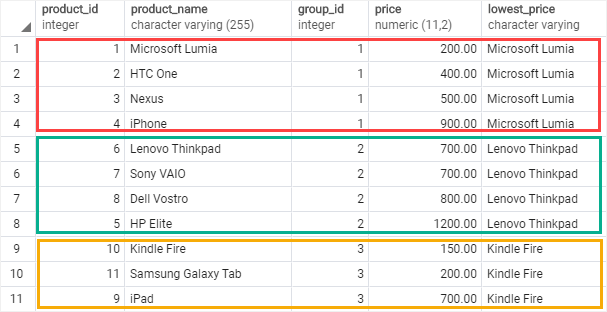# PostgreSQL Tutorial: FIRST_VALUE Function

August 7, 2023

Summary: in this tutorial, you will learn how to use the PostgreSQL `FIRST_VALUE()` function to return the first value in a sorted partition of a result set.

## Introduction to PostgreSQL `FIRST_VALUE()` function

The `FIRST_VALUE()` function returns a value evaluated against the first row in a sorted partition of a result set.

The following is the syntax of the `FIRST_VALUE()` function:

``````FIRST_VALUE ( expression )
OVER (
[PARTITION BY partition_expression, ... ]
ORDER BY sort_expression [ASC | DESC], ...

FIRST_VALUE ( expression )
OVER (
[PARTITION BY partition_expression, ... ]
ORDER BY sort_expression [ASC | DESC], ...
)
``````

In this syntax:

### `expression`

The `expression` can be an expression, column, or subquery evaluated against the value of the first row of a sorted partition of a result set. The `expression` must return a single value. And it cannot be a window function.

### `PARTITION BY` clause

The `PARTITION BY` clause divides rows in a result set into partitions to which the `FIRST_VALUE()` function is applied.

The `PARITION BY` clause is optional. If you skip it, the `FIRST_VALUE()` function treats the whole result set as a single partition.

### `ORDER BY` clause

The `ORDER BY` clause specifies the sort order of rows in each partition to which the `FIRST_VALUE()`function is applied.

### `rows_range_clause`

The `rows_range_clause` further limits the rows within the partition by defining the start and end in the partition

## PostgreSQL `FIRST_VALUE()` function examples

We will use the `products` table created in the window function tutorial for the demonstration:The data of the `products` table is as follows:### 1) Using PostgreSQL `FIRST_VALUE()` function over a result set example

The following statement uses the `FIRST_VALUE()` function to return all products and also the product which has the lowest price:

``````SELECT
product_id,
product_name,
group_id,
price,
FIRST_VALUE(product_name)
OVER(
ORDER BY price
) lowest_price
FROM
products;
``````

Here is the result set:In this example:

• Since we skipped the `PARTITION BY` clause in the `FIRST_VALUE()` function, the function treated the whole result set as a single partition.
• The `ORDER BY` clause sorted products by prices from low to high.
• The `FIRST_VALUE()` function is applied to the whole result set and picked the value in the `product_name` column of the first row.

### 2) Using `FIRST_VALUE()` function over a partition example

This statement uses the `FIRST_VALUE()` function to return all products grouped by the product group. And for each product group, it returns the product with the lowest price:

``````SELECT
product_id,
product_name,
group_id,
price,
FIRST_VALUE(product_name)
OVER(
PARTITION BY group_id
ORDER BY price
RANGE BETWEEN
UNBOUNDED PRECEDING AND
UNBOUNDED FOLLOWING
) lowest_price
FROM
products;
``````In this example:

• The `PARTITION BY` clause distributed products by product group.
• The `ORDER BY` clause sorted products in each product group (partition) by prices from low to high.
• The `RANGE BETWEEN UNBOUNDED PRECEDING AND UNBOUNDED FOLLOWING` clause defined the frame in each partition, starting from the first row and ending at the last row.
• The `FIRST_VALUE()` function is applied to each partition separately.

In this tutorial, you have learned how to use the PostgreSQL `FIRST_VALUE()` function to return the first value in a sorted partition of a result set.